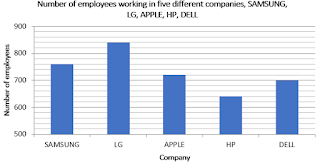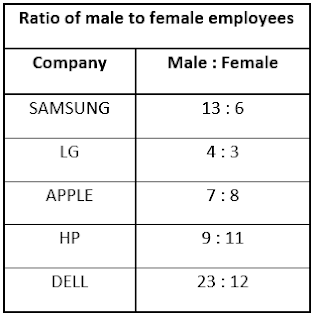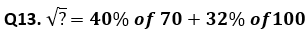Dear students,

Numerical Ability or Quantitative Aptitude Section has given heebie-jeebies to the aspirants when they appear for a banking examination. As the level of every other section is only getting complex and convoluted, there is no doubt that this section, too, makes your blood run cold. The questions asked in this section are calculative and very time-consuming. But once dealt with proper strategy, speed, and accuracy, this section can get you the maximum marks in the examination. Following is the Quantitative Aptitude quiz to help you practice with the best of latest pattern questions.

Q1. Two pipes can fill a tank in 10 hours and 16 hours respectively. A third pipe can empty the tank in 32 hours. If all the three pipes are opened simultaneously then in how much time the tank will be full? (in hours)

Q2. In a class the number of boys and number of girls are in the ratio of 2 : 3. If the number of boys is increased by 20% and number of girls is increased by 20 then the new ratio of the number of boys to that of girls is 3:5. What is the total strength after increase?
112
132
128
Data inadequate
None of these

Q3.Speed of a truck is 2/3rd of speed of a car. Truck takes 120 minutes more than car to cover a distance of 288 km. If speed of a train is 100% more than speed of truck, then find in what time train will cover a total distance of 576 km?
4 hours
3 hours
6 hours
8 hours
10 hours

Q4. The average age of all the students of a class is 16 years. The average age of boys of the class is 21 years and that of the girls is 12 years. What is the ratio of the boys to the girls?
4:5
2:3
1:2
1:3
4:7

Q5. The sum of the ages of father and son is 50 years. Eight years ago, the product of their ages was two time the father’s age at that time, then the present ages (in years) of the father and son respectively are
39,6
35,10
36,9
40,10
42, 8

Directions (6-10): Study the following bar diagram and table carefully to answer the questions:Q6. Number of females, employees in company Apple and Samsung together is approximately what percent more or less than the number of male employees in H.P and Dell.
12% more
17% less
18% more
20% less
20% more

Q7. If 10% male resign from L.G. and 50% female joins Apple then what will be the ratio of total Employee working in LG to the total employees working in Apple after changes?
33/38
34/37
33/17
35/31
None of these

Q8. What is the approx. difference between average number of males over all departments and average number of females over all departments.
100
150
102
110
120

Q9. If in another company xyz total number of employees are 90% of the total no. of employees over all departments and ratio of male to female in xyz is 1 : 2 then total number of males in xyz are what percent of total employees from company LG.
200%
150%
125%
131%
250%

Q10. Total number of males from Samsung and LG together are approximately what percent more than the number of females from company HP and Dell together?
60%
65%
69%
75%
85%

Directions (11 -15) Simplify the following questions and find the value of (?).

Q11. 5÷50 + 55 ÷ 5500 + 555 ÷ 555000 = ?
0.222
0.111
0.125
0.134
0.452

Q12. 276.3 + 343.8 + 545.3 –873.4 = ?
292
397
289
277
281
Solution:
? = 2923844
3424
3600
4024
3244Q15. 15 × 6 + 75 – 95 =? + 40
25
35
30
40
50

You May also like to Read: